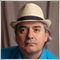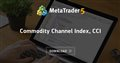# CCI Mathematically10

 We all know the mq4 code for the CCI....double  iCCI(string symbol, int timeframe, int period, int applied_price, int shift);

And this is the mathematics formula, Value of CCI = (Typical Price - Xperiod SMA of TP) / (0.015 x Mean Deviation)

However, how would you write it using the mathematical functions of mq4.... I have no idea, but would like to use parts of the mathematical formula to look at different uses of the Commodity Channel Index. Any help would be much appreciated.5705

4aCause:
 We all know the mq4 code for the CCI....double  iCCI(string symbol, int timeframe, int period, int applied_price, int shift);

And this is the mathematics formula, Value of CCI = (Typical Price - Xperiod SMA of TP) / (0.015 x Mean Deviation)

However, how would you write it using the mathematical functions of mq4.... I have no idea, but would like to use parts of the mathematical formula to look at different uses of the Commodity Channel Index. Any help would be much appreciated.

Have a look at the following in the CodeBase as it is the basis of the iCCI() function in MQL4.Commodity Channel Index, CCI
• www.mql5.com
Commodity Channel Index (CCI) indicator measures the deviation of the commodity price from its average statistical price.10

You're a gem Fernando. Thank you very much!!